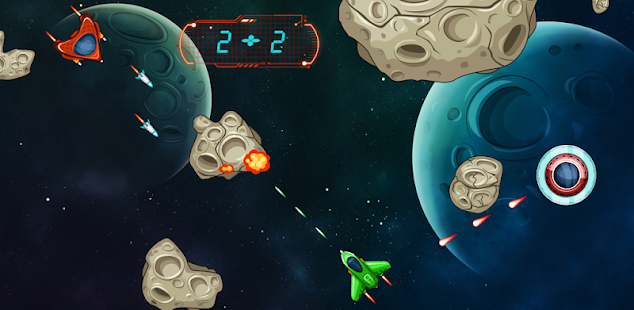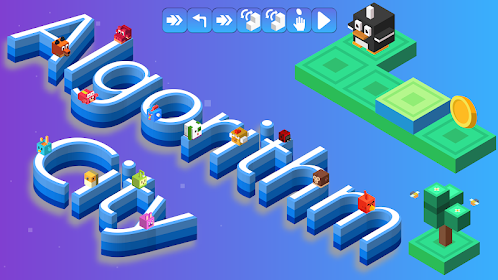The Math Space HeroEveryone
600The Math Space Hero Game is a funny math game. In this game you find yourself in a space ship, trying to escape the meteors, UFOs, enemies and planets. At the same time you have to shoot the solutions of appearing at the top of green field tasks.
You may see all feedback responses at the end of the game. Feedback is available for all questions.
There are 8 class levels in the game:

1st class level -> used the numbers 1-20
* Subtraction
* The largest number
* The smallest number
* The next number in simple patterns

2nd class level -> used the numbers 1-20
In addition to 1st class level
* Multiplication
* Division
* Addition and subtraction with three number
* The result of the addition operations
* The result of the subtraction operations

3rd class level -> used the numbers 1-200
In addition to 2nd class level
* The even number
* The odd number
* The next number in patterns
* The result of the multiplication operations
* The result of the division operations

4th class level -> used the numbers 1-500
In addition to 3rd class level
* The result of the mixed operations

5th class level -> The numbers from 1 to 1000 this level had been used.
In addition to 4th class level
* The square of number 1 to 20
* 1 to find the cubes of natural numbers with up to 6
* Finding the number is not given in Patterns

6th class level -> used the numbers 1-1000
In addition to 5th class level
* the square of number 1-10
* Prime numbers between 1 and 50
* Subtraction of algebraic expressions

7th class level -> used the numbers -1000 to 1000
In addition to 6th class level
* Also used negative numbers
* Prime numbers between 1 and 100
* Multiplication of algebraic expressions

8th class level-> used the numbers -1000 to 1000
In addition to 7th class level
* Square root of numbers
* Subtraction of Irrational numbers
Collapse

Reviews

Review policy and info
3.9
600 total
5
4
3
2
1

What's New

Fixed some bugs.
Question difficulty level has been updated.
Collapse

Updated
October 20, 2017
Size
16M
Installs
100,000+
Current Version
1.1.0
Requires Android
2.3 and up
Content Rating
Everyone
Violent References
Permissions
Offered By
Musteren
Developer
İçerenköy M. Karslı Ahmet C. Özyurt S.No:24/0 ATAŞEHİR/İSTANBUL/TÜRKİYE